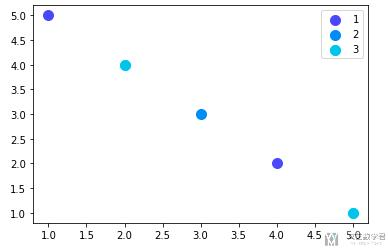# matplotlib绘图优化-散点图绘制图例2020年6月15日07:07:40

1473字阅读4分54秒

## 简单实验

1. import numpy as np
2. from matplotlib import pyplot as plt

1. scatter_x = np.array([1,2,3,4,5])
2. scatter_y = np.array([5,4,3,2,1])
3. group = np.array([1,3,2,1,3])
4. cdict = {1: 'red', 2: 'blue', 3: 'green'}

1. fig, ax = plt.subplots()
2. for g in np.unique(group):
3.     ix = np.where(group == g)
4.     ax.scatter(scatter_x[ix], scatter_y[ix], c = cdict[g], label = g, s = 100)
5. ax.legend()
6. plt.show()

1. for key in cdict.keys():
2.     ix = np.where(group == key)
3.     ax.scatter(scatter_x[ix], scatter_y[ix], c = cdict[key], label = key, s = 100)

### 关于散点图的颜色

1. cmap = plt.get_cmap('rainbow', 12) # 数字与颜色的转换

1. cmap = plt.get_cmap('rainbow', 12) # 数字与颜色的转换
2. scatter_x = np.array([1,2,3,4,5])
3. scatter_y = np.array([5,4,3,2,1])
4. group = np.array([1,3,2,1,3])
5. cdict = {1: '111', 2: '222', 3: '333'}
6. fig, ax = plt.subplots()
7. for key in cdict.keys():
8.     ix = np.where(group == key)
9.     ax.scatter(scatter_x[ix], scatter_y[ix], color = cmap(key), label = key, s = 100) # 注意这里color的指定
10. ax.legend()
11. plt.show()• 微信公众号
• 关注微信公众号
•• QQ群
• 我们的QQ群号
•• 本文由 发表于 2020年6月15日07:07:40
• 转载请务必保留本文链接：https://mathpretty.com/12395.html